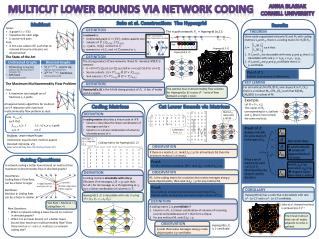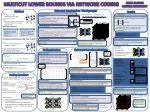Download PresentationI M

# I M - PowerPoint PPT Presentation

Multicut lower bounds via network coding. Anna Blasiak Cornell University. Multicut. Results. Given: A graph G = (V,E) C apacities for each edge k source-sink pairs Find: A min-cost subset of E such that on removal all source-sink pairs are disconnected.I am the owner, or an agent authorized to act on behalf of the owner, of the copyrighted work described.
Download Presentation## I M

An Image/Link below is provided (as is) to download presentation

Download Policy: Content on the Website is provided to you AS IS for your information and personal use and may not be sold / licensed / shared on other websites without getting consent from its author.While downloading, if for some reason you are not able to download a presentation, the publisher may have deleted the file from their server.

- - - - - - - - - - - - - - - - - - - - - - - - - - E N D - - - - - - - - - - - - - - - - - - - - - - - - - -
Presentation TranscriptMulticut lower bounds via network coding

Anna Blasiak

Cornell University

Multicut

Results

• Given:
• A graph G = (V,E)
• Capacities for each edge
• k source-sink pairs
• Find:
• A min-cost subset of E such that on removal all source-sink pairs are disconnected

The n-path network Pn= Hypergrid(n,1):

s

s

s

s

s

s

Node capacitated ⊆ Edge capacitated, directed

1

1

Key

1

S1T1S2T2S3T3

1

Hypergrid(3, 3)

Hypergrid(3, 2)

s2

s2

s2

Flow Rate = Multicut = 1, Coding Rate = k

The optimal max multicommodity flow solution for Hypergrid(n, k)routes nk-1units of flow between a single s-t pair.

The trivial multicut that cuts all nodes adjacent to sinks is optimal.

s2

1

1

Theorem

definition

observation

observation

Observation

definition

definition

Definition

Definition

definition

Key lemma

t

t

Corollary

The State of the Art

t

t

t

t

(v1 , v1’ )

(v1 , v1’ )

(v1 , v1’ )

(v1 , v1’ )

(v2 , v1’ )

(v2 , v1’ )

(v2 , v1’ )

(v2 , v1’ )

(v3, v1’ )

(v3, v1’ )

(v3, v1’ )

(v3, v1’ )

Given node-capacitated networks N1and N2 with coding matrices L1and L2, there is a coding matrix for N1☒ N2 :

L =such that:

If L1and L2 are decodable with rates p1and p2 then L is decodable with rate p := n1p2 + n2 p1- p1p2.

If L1and L2 are p1and p2 certifiable then Lis p-certifiable.

For all multicutsMof N1☒N2 and cliques Kin V1 (V2)there is a multicutMK of N2(N1)such that K⊗MK(MK⊗K)is a subset of M.

• The strong product of two networks N and N’, denoted N ☒N’, is network:
• G = ( V×V’, {((u,u’), (v,v’)) |(u,v)∈E oru=v, (u’,v’)∈E’oru’=v’})
• Source subsets: {Si × V’}i∈[k]U{V × S’i}i∈[k’]
• Sink subsets: {Ti × V’}i∈[k]U{V × T’i}i∈[k’]

A coding matrix is decodable with rate pif∃subsetDof messages, |D|= p, such that:

For all i, for all message mi∈ D originating at si, miis a linear combination of columns in Ti.

• A network is:
• Undirected graph G = (V,E ), nodes capacity one
• Subsets of V: {Si}i∈[k], {Ti}i∈[k]
• si - tipairs, i∈[k], connect to G:
• siconnects to v ∈Si , and v ∈Ti connect to ti

Hypergrid(n, k)is the k-fold strong product of Pn. It has nknodes and k s-t pairs.

A coding matrix L is p-certifiable if

Column vof L is a linear combination of columns of incoming sources and predecessors of vthat form a clique.

For any multicutM,rank(LIM)≥ p.

Hypergrid(n,k) has a code that is decodable with rate nk- (n-1)kand is nk- (n-1)k certifiable.

t1

t1

t1

t1

s1

s1

s1

s1

(v2 , v2’ )

(v1 , v2’ )

(v1 , v2’ )

(v1 , v2’ )

(v1 , v2’ )

(v2 , v2’ )

(v2 , v2’ )

(v2 , v2’ )

(v3, v2’ )

(v3, v2’ )

(v3, v2’ )

(v3, v2’ )

s1

t1

v1 v2 v3 v4 v5 vn-1 vn

(v1 , v3’ )

(v1 , v3’ )

(v1 , v3’ )

(v1 , v3’ )

(v2 , v3’ )

(v2 , v3’ )

(v2 , v3’ )

(v2 , v3’ )

(v3, v3’ )

(v3, v3’ )

(v3, v3’ )

(v3, v3’ )

Proof of 1

A direct consequence of definitions.

t2

t2

t2

t2

The Maximum Multicommodity Flow Problem

• Find:
• A maximum total weight set of fractional si-ti paths.

Example:

Let K = {v1, v2}.

The copies of N2 corresponding to v1 (yellow) and v2 (blue) must contain the same multicut.

• All approximation algorithms for multicut use LP relaxation with maximum multicommodity flow problem as dual.

Coding Matrices

Cut Lower Bounds via Matrices

Matrix IM represents a cut M.

IM

min Σein E xe

such that

Σein p xe≥ 1 1 ≤i≤ k, p si-tipath

xe≥ 0 e ∈ E

L

• A coding matrix describes a linear code of N if:
• Column v describes the linear combination of messages sent by v
• Column vis a linear combination of columns of predecessors of v.

Saks et al. Construction: The Hypergrid

Proof of 2:

Analyze rank(LB) for some matrix B in the span of IM.

vijabbreviation for (vi , vj’ )

IMK

If L is the coding matrix for a solution that routes messages along p node-disjoint paths, then rank (LIM) ≥ p for any multicutM.

If there is a matrix Ls.t. rank(LIM)≥ p for all multicutsM, then the minimum multicutis at least p.

A code that routes messages along p node-disjoint paths is p-certifiable.

Problem: Large integrality gap

Undirected: equal to best multicutapprox

Directed: Ω(min((k, nδ)) [Saks, Samorodnitsky, Zosin ’04, Chuzhoy, Khanna’09 ]

Main proof ideas:

0

Messages ai, bi , cioriginate at si

Coding matrix for Hypergrid(3, 2)

0

K used to decode certain off diagonal blocks of LB vanish.

IMK

in K in V1

v4

B has a set of columns for each v ∈ V1U V2determined by the cliqueKused to decode v.

Guiding Questions

Proof:

For M a minimum multicut, |M| = rank (IM) ≥ rank (LIM) ≥ p.

rank(L2IMK)=p2

and rank(L1IMK’)=p1diagonal blocks have ranks p1and p2

Is network coding a better lower bound on multicutthan maximum multicommodity flow in directed graphs?

Good News:

Coding Rate ≥ Flow Rate,

can be a factor k larger

Multicut≱ Coding Rate,

can be a factor k smaller

IMK

capacity 1

All other edges have infinite capacity

+ edges between si and tjfor all i ≠ j

Proof:

M a multicut: at least one non-zero in each row of LIM

Disjoint paths: at most one non-zero in each column of LIM

Hypergrid(3, 2)is decodable with rate 5 using D = {a1, b1 , c1 , a2, b2 }.

v1

Saks et al. showed multicut is at leastk(n-1)k-1

• New Questions:
• When is network coding a lower bound on multicut in directed graphs?
• When it is an lower bound, is it a better lower bound than maximum multicommodity flow? Does there exist an α = o(k) s.t.multicut ≤ α network coding rate?

v’1

v’1

v’1

v’1

v’1

v2

v’n2

v’n2

v’n2

v’n2

v’n2

a1+a2

a1+b2

a1

v3

b1+a2

b1+b2

b1

Hypergrid(n,1) is 1-certifiable.

c1+a2

c1+b2

c1

vn1